### The Square Root of Two

June 6, 2016

As an undergraduate, I encountered quite a few interesting people from among both students and professors. There was the economics professor who wore the same necktie with a graph paper motif every day. There was the physics graduate student teaching assistant who began our first session by placing a trash can on his desk and saying, "elephant." He explained that was his way of ensuring that he would be remembered. He was right.

One of my student acquaintances was so comical, he should have made a career as a late night television host, and not as an engineer. One of his routines was to mimic the lectures of a certain professor. He would write furiously on a blackboard, stop, tap the chalk on a particular equation, look towards his audience, and exclaim, "Take square root... Take square root!"

The square root of two (1.4142135623..., sequence no. A002193 in the On-Line Encyclopedia of Integer Sequences) is an irrational number; that is, it can't be expressed as a ratio of integers. The integers include the natural numbers.

Early mathematicians considered negative numbers to be absurd. For that reason, all their fractions involved ratios of natural numbers, only. While the word, "absurd," means irrational, "surd" is actually a mathematics term relating to the roots of equations.Thales (624-546 BC, top) and Pythagoras (570-495 BC, bottom) were among the earliest mathematicians.

Thales is known for Thales' theorem that any angle inscribed in a semicircle is a right angle. He is also reported to have measured the height of the Egyptian pyramids using the concept of 45° similar triangles.

Pythagoras is known, of course, for the Pythagorean theorem. He invented the geometrical object known as the Tetractys.

(Thales image (top), and Pythagoras image (bottom), from the Nuremberg chronicle, via Wikimedia Commons.)

Natural numbers are called that for the simple reason that they are the counting numbers. What can be more natural than looking out a window and thinking, "One tree, two trees, three trees...?" The German mathematician Leopold Kronecker, who is known to scientists and engineers for his Kronecker delta function, and among mathematicians for giving Georg Cantor such a hard time, once said that "God made the integers, all else is the work of man."

Pythagoras made the discovery that musical instrument strings having integral fraction lengths were most harmonious. Instruments with Pythagorean tuning have tones anchored at a perfect fifth in which the G-note string in a C-major scale is 2/3 the length of the C-string. The modern evolution of this concept is just intonation, where all the notes are ratios of small natural numbers, as seen in the table.

 C D E F G A B 1/1 9/8 5/4 4/3 3/2 5/3 15/8

The followers of Pythagoras, the Pythagoreans, believed that everything in the universe could be related to the natural numbers and their ratios; so, it came as quite a surprise when Hippasus (c. 450 BC) showed that the length of the hypotenuse of an isosceles right triangle could not be expressed as a ratio of natural numbers. When the sides of such a triangle are of length one, this means that the square root of two is irrational. There's a legend that the gods caused Hippasus to be drowned at sea as punishment for this revelation.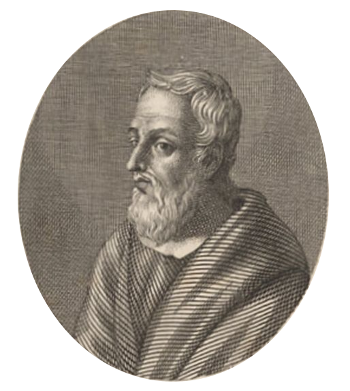Hippasus of Metapontum

Not much is known about Hippasus, other than his being a Pythagorean.

The years of his birth and death are not known, although it's likely that he lived in the 5th century BC, about a century after Pythagoras.

(Engraving, circa 1820, by G. Boccanera, via Wikimedia Commons.)

The method of proof employed by Hippasus is known as reductio ad absurdum. In this method, a proposition is shown to have two incompatible qualities, such as a number being both odd and even. This proof in the context of Hippasus's triangle is tedious, so it won't be explained here. You can read it on Wikipedia. I'll give a proof in modern notation, which is easier to understand.

First, we suppose that the square root of two really is rational, so it can be written as the ratio of two integers, p and q. We suppose the fraction has been reduced by canceling all common factors of p and q.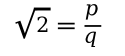We then square both sides of the equation.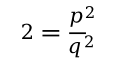Rearranging the equation, we see that p2 must be an even number.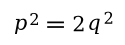If p2 is even, then p is even. You can see that this is true, since having a 1, 3, 5, 7, or 9 as the last digit of p would give 1, 9, 5, 9, or 1 as the last digit of p2. If p is even, then p2 is divisible by four, so q2 and q must be even.

Now comes the reductio ad absurdum part. We showed that both p and q are even, which contradicts our requirement that the initial fraction was properly reduced. Since our analysis gives this false result, then our initial assumption that the square root of two is rational is also false.

The date for the Greek proof of the irrationality of the square root of two is about 450 BC. The Greeks, however, weren't the only people doing mathematics in antiquity. As I wrote in a recent article (Mathematical Astronomy in Babylon, March 10, 2016), the Babylonians were adept at the use of mathematics in astronomy from a very early date.

Benjamin M. Altschuler of the The Fieldston School (Bronx, New York), and Eric L. Altschuler of the Lewis Katz School of Medicine at Temple University (Philadelphia, Pennsylvania), have just presented evidence that a clay tablet from the Old Babylonian Empire (20th - 16th centuries BC) may contain a geometrical construction leading to a proof of the irrationality of the square root of two similar to that of Hippasus.

This tablet, known as BM 15285, contains 41 geometrical area problems. Problem twelve, as shown in the figure, has the following associated text: "The side of the square is 60 rods. Inside it [I drew]16 wedges [triangles]. What are their areas?" The figure does not accompany an explicit proof of the irrationality of the square root of two, but the authors explain that its essential elements are there. See the arXiv paper for details.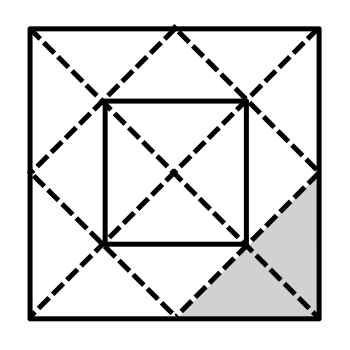Problem xii of tablet BM15285.

The shaded area is a missing part of the tablet.

(Drawn using Inkscape.)### References:

1. "Die ganzen Zahlen hat der liebe Gott gemacht, alles andere ist Menschenwerk."
2. Benjamin M. Altschuler and Eric L. Altschuler, "Proof of the Irrationality of the Square Root of Two in Babylonian Geometry Problem Tablets," arXiv, March 22, 2016.
3. Images of Babylonian tablet BM15285, PDF file at the University of Helsinki.

Linked Keywords: Undergraduate; student; professor; economics; necktie; graph paper; motif; physics; postgraduate education; graduate student; teaching assistant; waste container; trash can; desk; elephant; comedy; comical; career; late night television host; engineer; sketch comedy; routine; impressionist; mimic; lecture; blackboard; chalk; equation; audience; square root; square root of two; A002193; On-Line Encyclopedia of Integer Sequences; irrational number; ratio; integer; natural numbers; mathematician; negative number; absurd; surd; Thales; Pythagoras; Thales' theorem; angle; semicircle; right angle; Egyptian pyramids; similar triangles; Pythagorean theorem; geometry; geometrical; Tetractys; Nuremberg chronicle; Wikimedia Commons; counting numbers; nature; natural; window; tree; German; Leopold Kronecker; scientist; engineer; Kronecker delta function; Georg Cantor; God; man; musical instrument; string; fraction; harmony; harmonious; Pythagorean tuning; musical note; tone; perfect fifth; G-note string; C-major scale; just intonation; musical note C; musical note D; musical note E; musical note F; musical note G; musical note A; musical note B; Pythagoreanism; Pythagorean; universe; Hippasus (c. 450 BC); hypotenuse; isosceles triangle; right triangle; legend; deity; god; drowning; drown; sea; punishment; Metapontum; 5th century BC; century; mathematical proof; reductio ad absurdum; number; odd; even; Ancient Greece; irreducible fraction; reduce; factorization; common factors; square; divisor; divisible; Babylonia; Babylonians; The Fieldston School (Bronx, New York); Eric L. Altschuler; Lewis Katz School of Medicine at Temple University (Philadelphia, Pennsylvania); clay tablet; Old Babylonian Empire; area; square; rod; arXiv; Inkscape.